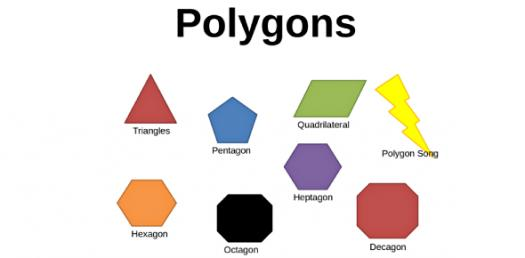# Polygon, Pentagon And Decagon: Interior And Exterior Angle Sum! Trivia Quiz

11 Questions | Total Attempts: 210SettingsIn this quiz, we get to review the Polygon, Pentagon, and Decagon: Interior and Exterior Angle Sum! Most students have had a hard time when it comes to some of the solutions when it comes to these shapes. Are you one of them? Do give this quiz a try and be a step closer to understanding how to find some of the angles and their relations.

• 1.
How many sides does a nonagon have?
• A.

5

• B.

6

• C.

7

• D.

8

• E.

9

• 2.
Does a regular polygon have I. congruent sides II. congruent angles III. dents
• A.

I only

• B.

II only

• C.

I and II only

• D.

I, II and III

• E.

III only

• 3.
How many sides does the structure of The Pentagon have?
• A.

5

• B.

6

• C.

7

• D.

8

• E.

9

• 4.
The figure below can be described by which of the following? I. regular polygon II. convex polygon III. hexagon
• A.

I only

• B.

II only

• C.

III only

• D.

I and II only

• E.

I, II and III

• 5.
What is the interior angle sum of a hexagon in degrees?
• A.

180

• B.

360

• C.

540

• D.

720

• E.

900

• 6.
How many sides does a convex polygon have if the interior angle sum is 540 degrees?
• A.

4

• B.

5

• C.

6

• D.

7

• E.

8

• 7.
What is the measure in degrees of each interior angle of a regular do-decagon?
• A.

150

• B.

135

• C.

144

• D.

108

• E.

120

• 8.
What is the measure in degrees of each interior angle of a regular pentagon?
• A.

135

• B.

144

• C.

120

• D.

108

• E.

150

• 9.
What is the exterior angle sum of any polygon?
• A.

180

• B.

270

• C.

360

• D.

540

• E.

720

• 10.
One exterior angle of a regular polygon is equal to 20 degrees. How many sides does it have?
• A.

9

• B.

10

• C.

12

• D.

16

• E.

18

• 11.
What is the measure in degrees of each exterior angle of a regular decagon?
• A.

20

• B.

36

• C.

10

• D.

18

• E.

35

Related Topics